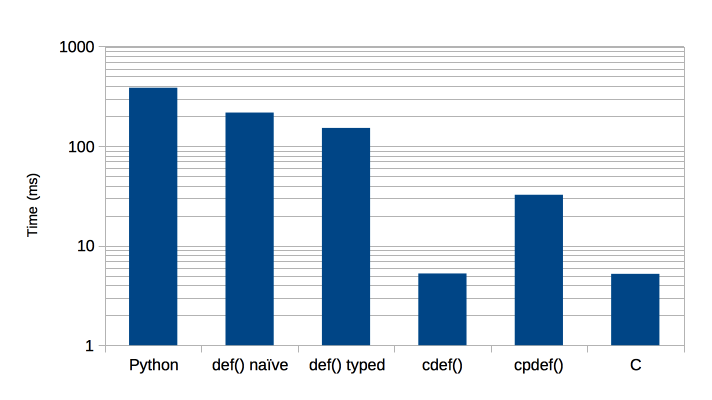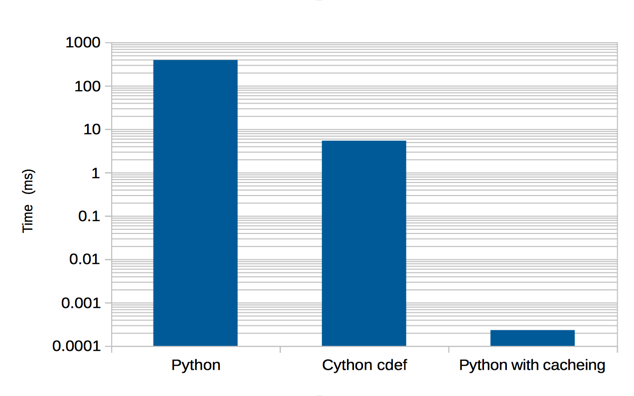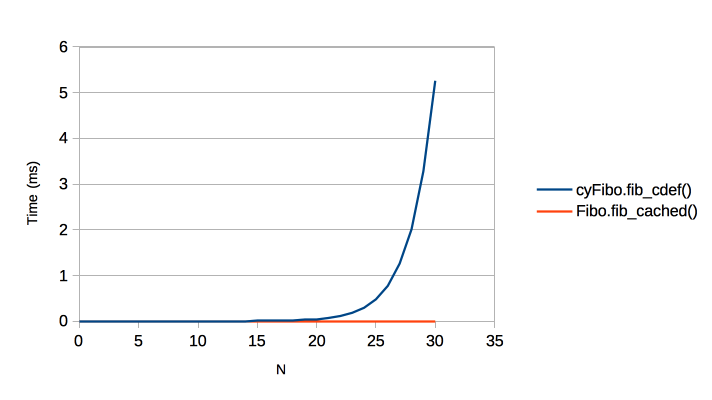# How Fast are `def``cdef``cpdef`?¶

## Code Example¶

Here is an example of computing the Fibonacci series (badly) that will be done in Python, Cython and C.

First up, Python [Fibo.py]:

```def fib(n):
if n < 2:
return n
return fib(n-2) + fib(n-1)
```

In naive Cython [cyFibo.pyx], it is the same code:

```def fib(n):
if n < 2:
return n
return fib(n-2) + fib(n-1)
```

Optimised Cython where we specify the argument type [cyFibo.pyx]:

```def fib_int(int n):
if n < 2:
return n
return fib_int(n-2) + fib_int(n-1)
```

In Cython calling C generated code. Here we use a `def` to call a `cdef` that does the body of the work [cyFibo.pyx]:

```def fib_cdef(int n):
return fib_in_c(n)

cdef int fib_in_c(int n):
if n < 2:
return n
return fib_in_c(n-2) + fib_in_c(n-1)
```

Now a recursive `cpdef`:

```cpdef fib_cpdef(int n):
if n < 2:
return n
return fib_cpdef(n-2) + fib_cpdef(n-1)
```

Finally a C extension. We expect this to be the fastest way of computing the result given the algorithm we have chosen:

```#include "Python.h"

/* This is the function that actually computes the Fibonacci value. */
static long c_fibonacci(long ord) {
if (ord < 2) {
return ord;
}
return c_fibonacci(ord - 2) + c_fibonacci(ord -1);
}

/* The Python interface to the C code. */
static PyObject *python_fibonacci(PyObject *module, PyObject *arg) {
PyObject *ret = NULL;
assert(arg);
Py_INCREF(arg);
if (! PyLong_CheckExact(arg)) {
PyErr_SetString(PyExc_ValueError, "Argument is not an integer.");
goto except;
}
long ordinal = PyLong_AsLong(arg);
long result = c_fibonacci(ordinal);
ret = PyLong_FromLong(result);
assert(! PyErr_Occurred());
assert(ret);
goto finally;
except:
Py_XDECREF(ret);
ret = NULL;
finally:
Py_DECREF(arg);
return ret;
}

/********* The rest is standard Python Extension code ***********/

static PyMethodDef cFiboExt_methods[] = {
{"fib", python_fibonacci, METH_O, "Fibonacci value."},
{NULL, NULL, 0, NULL}           /* sentinel */
};

#if PY_MAJOR_VERSION >= 3

/********* PYTHON 3 Boilerplate ***********/

PyDoc_STRVAR(module_doc, "Fibonacci in C.");

static struct PyModuleDef cFiboExt = {
"cFibo",
module_doc,
-1,
cFiboExt_methods,
NULL,
NULL,
NULL,
NULL
};

PyMODINIT_FUNC
PyInit_cFibo(void)
{
return PyModule_Create(&cFiboExt);
}

#else

/********* PYTHON 2 Boilerplate ***********/

PyMODINIT_FUNC
initcFibo(void)
{
(void) Py_InitModule("cFibo", cFiboExt_methods);
}

#endif
```

## Benchmarks¶

First a correctness check on Fibonacci(30):

```\$ python3 -c "import Fibo, cyFibo, cFibo; print(Fibo.fib(30) == cyFibo.fib(30) == cyFibo.fib_int(30) == cyFibo.fib_cdef(30) == cyFibo.fib_cpdef(30) == cFibo.fib(30))"
True
```

Now time these algorithms on Fibonacci(30) thus:

```\$ python3 -m timeit -s "import Fibo" "Fibo.fib(30)"
\$ python3 -m timeit -s "import cyFibo" "cyFibo.fib(30)"
\$ python3 -m timeit -s "import cyFibo" "cyFibo.fib_int(30)"
\$ python3 -m timeit -s "import cyFibo" "cyFibo.fib_cdef(30)"
\$ python3 -m timeit -s "import cyFibo" "cyFibo.fib_cpdef(30)"
\$ python3 -m timeit -s "import cFibo" "cFibo.fib(30)"
```

Gives:

Language Function call Time (ms) Speed, Python = 1
Python `Fibo.fib(30)` 390 x 1
Cython `cyFibo.fib(30)` 215 x 1.8
Cython `cyFibo.fib_int(30)` 154 x 2.5
Cython `cyFibo.fib_cdef(30)` 5.38 x72
Cython `cyFibo.fib_cpdef(30)` 32.5 x12
C `cFibo.fib(30)` 5.31 x73

Graphically:The conclusions that I draw from this are:

• Naive Cython does speed things up, but not by much (x1.8).
• Optimised Cython is fairly effortless (in this case) and worthwhile (x2.5).
• `cdef` is really valuable (x72).
• `cpdef` gives a good improvement over `def` because the recursive case exploits C functions.
• Cython’s `cdef` is insignificantly different from the more complicated C extension that is our best attempt.

## The Importance of the Algorithm¶

So far we have looked at pushing code into Cython/C to get a performance gain however there is a glaring error in our code. The algorithm we have been using is very inefficient. Here is different algorithm, in pure Python, that will beat all of those above by a huge margin :

```def fib_cached(n, cache={}):
if n < 2:
return n
try:
val = cache[n]
except KeyError:
val = fib(n-2) + fib(n-1)
cache[n] = val
return val
```

And timing it for Fibonacci(30) gives:

Language Function call Time (ms) Improvement
Python `Fibo.fib(30)` 390 x1
Cython `cyFibo.fib_cdef(30)` 5.38 x72
Python `Fibo.fib_cached(30)` 0.000231 x1.7e6

Or, graphically:In fact our new algorithm is far, far better than that. Here is the O(N) behaviour where N is the Fibonacci ordinal:Hammering a bad algorithm with a fast language is worse than using a good algorithm and a slow language.

Footnotes

  If you are using Python3 you can use the `functools.lru_cache` decorator that gives you more control over cache behaviour.